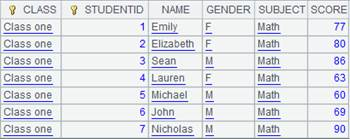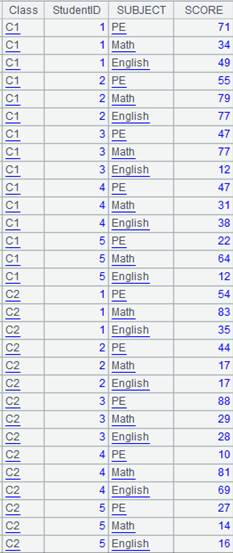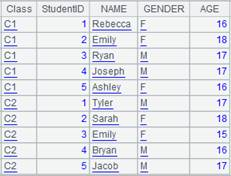# 循环函数中的表达式书写规则

Ø  基成员：~A.~~特指当前正在处理的记录。

 A 1 =demo.query("select * from EMPLOYEE") 2 =A1.(age(~.HIREDATE)) [9,6,3,7,9,8,…] 3 =A1.new(~.NAME:Name,age(~.HIREDATE):Workage)Ø  序号：#A.##特指基成员的次序号Ø  字段：F~.Fr.FA.F 相当于A.~.F不循环时A.F相当于A(1).FØ  A[i]~[i]，在循环中，相当于A(#+i)，即从A的当前成员往后数，

Ø  i个成员返回，越界不报错，返回nullF[]则相当于A[].F

 A 1 =demo.query("select ORDERID,AMOUNT,'' as ACCUMULATION from SALES") 2 =A1.run(ACCUMULATION=AMOUNT+ACCUMULATION[-1]) 用上一条记录的ACCUMULATION加当前的AMOUNT，得到当前记录的ACCUMULATION

Ø  A[a,b]~[a,b]，在循环中，以A(#+a)为起始值，以 A(#+b).为结束值，

Ø  返回这个区间的成员组成的序列。a缺省为1-#b缺省为A.len()-#

 A 1 =demo.query("select STOCKID,DATE,CLOSING,'' as FirstThree from STOCKRECORDS") 2 =A1.run(FIRSTTHREE=~[-3,-1].(CLOSING).avg()) ~[-3,-1]表示当前行往前数三条记录组成的集合

Ø  迭代：A1.f1(A2.f2(x))

x中的符号将优先解释为从属于A2 如果x从属于A1要写成A1.f1(A2.f2(A1.x))

 A 1 =demo.query("select * from EMPLOYEE") 2 =demo.query("select * from DEPARTMENT") 3 =A2.run(MANAGER=A1.select@1(EID==A2.~.MANAGER).NAME) 对循环嵌套的引用

Ø  get(level,F;a:b)

 A 1 [1,2,3] 2 =A1.(A1.(abs(~-get(1)))) 计算每个值与其他值的最大距离 3 =demo.query("select * from employee") 4 =A3.derive(A3.max(abs(SALARY-get(1,SALARY))):MaxDiff) 计算当前工资和其他人的最大工资差